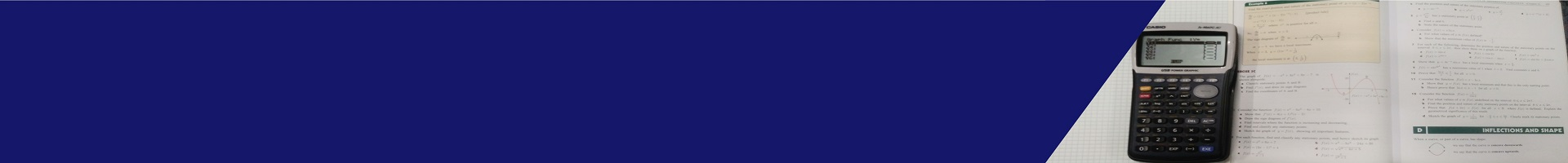# Mathematical Methods

#### Teacher: Brock Rogers

Mathematical Methods develops an increasingly complex and sophisticated understanding of calculus, statistics, mathematical arguments, and proofs, and using mathematical models.

By using functions, their derivatives, and integrals, and by mathematically modelling physical processes, students develop a deep understanding of the physical world through a sound knowledge of relationships involving rates of change. Students use statistics to describe and analyse phenomena that involve uncertainty and variation.

Topics for Semester 1 include Functions and Graphs, Polynomials and Trigonometry.

Semester 2 focuses on Counting, Introductory Calculus and Growth and Decay.

#### Assessment:

• Assessment Type 1: Skills and Applications Tasks (75%)
• Assessment Type 2: Folio (25%)

#### Pathways:

Mathematical Methods provides the foundation for further study in mathematics in Stage 2 Mathematical Methods and Stage 2 Specialist Mathematics.